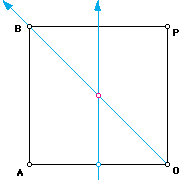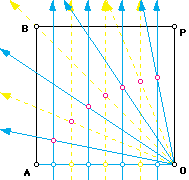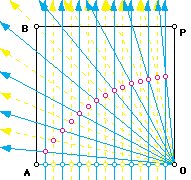# True Construction of Points on Hippias' QuadratrixAlthough Hippias' quadratrix cannot truely be constructed in the classical Greek sense of construction - i.e. compass and straightedge - we can classically construct 2^n points on the curve for arbitrarily large n. This construction is giving in the following activity.

1. Start by constructing a square ABPO.
2. Bisect angle AOP.
3. Construct the perpendicular bisector of segment OA.
4. The point of intersection of the angle bisector and the perpendicular bisector is a point on the quadratrix.
5. To construct two additional points on the quadratrix, bisect the two angles formed in Step 1.
6. Construct the perpendicular bisectors of the two segments formed in Step 2 (by bisecting OA).
7. The intersection of corresponding angle bisectors and perpendicular bisectors are points on the quadratrix.
8. Continue in this manner by dividing angle AOP into 2^n parts and segment OA into the same number of parts for arbitrarily large n. The intersection of corresponding parts are points on the quadratrix.It is interesting to note that this construction method to find points on Hippias' quadratrix has nothing to do with the concept of uniform motion in the difinition.